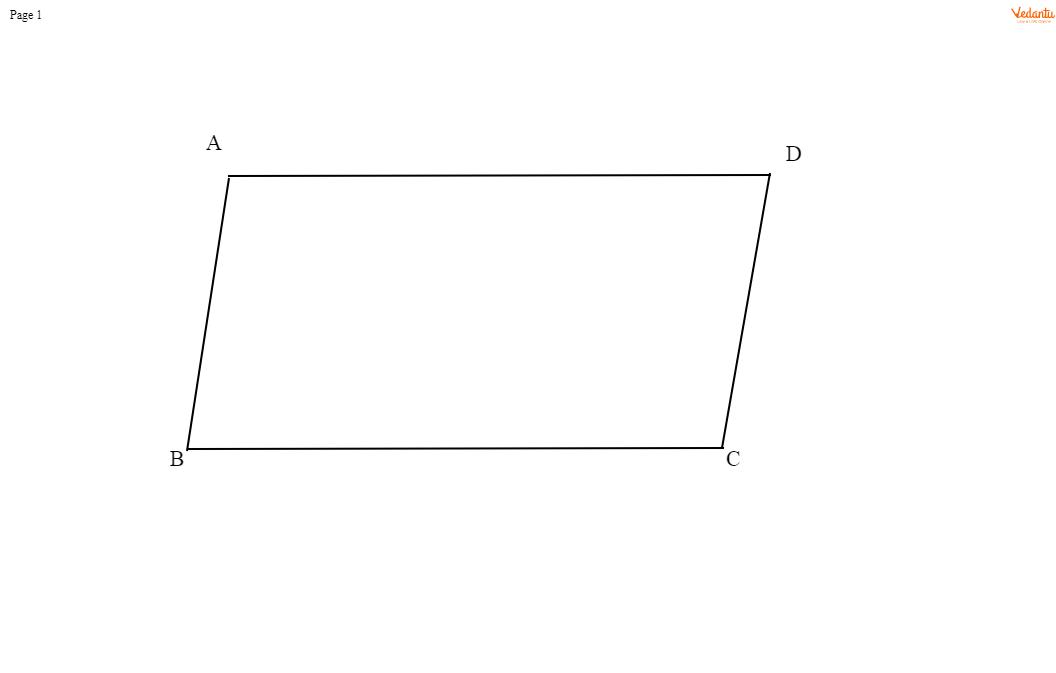QuestionAnswers

# Sum of the adjacent angles of a parallelogram is equal toA) 60\$^\circ \$B) 90\$^\circ \$C) 150\$^\circ \$D) 180\$^\circ \$Verified
113.4K+ Views
Hint- In this particular type of question, we need to draw a parallelogram and understand its geometry to write the desired answer. Using the properties of parallelograms we can find the solution for this problem.